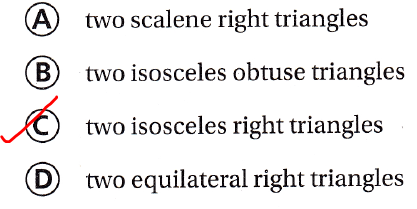Refer to our Texas Go Math Grade 5 Answer Key Pdf to score good marks in the exams. Test yourself by practicing the problems from Texas Go Math Grade 5 Lesson 11.2 Answer Key Triangles.

Essential Question
How can you classify triangles?
Triangles can be classified either according to their sides or according to their angles. All of each may be of different or the same sizes; any two sides or angles may be of the same size; there may be one distinctive angle
Now,
According to the sides, the triangles are classified into:
a. Equilateral triangle
b. Isosceles triangle
c. Scalene triangle
According to the angles, the triangles are classified into:
a. Acute triangle
b. Right triangle
c. Obtuse triangle

Unlock the Problem
If you look closely at Epcot Center’s Spaceship Earth building in Orlando, Florida, you may see a pattern of triangles. The triangle outlined in the pattern at the right has 3 congruent sides and 3 acute angles. What type of triangle is outlined?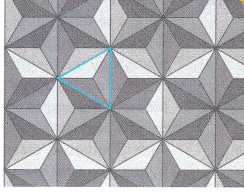Complete the sentence that describes each type of triangle.

Classify triangles by the lengths of their sides.

An equilateral triangle has 3 congruent sides.An isosceles triangle has 2 congruent sides.A scalene triangle has no congruent sides.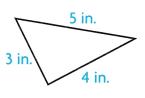Classify triangles by the measures of their angles.

A right triangle has one 90° or one right angle.An acute triangle has 3 acute angles.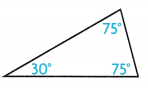An obtuse triangle has 1 obtuse angle.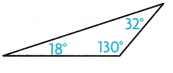The type of triangle outlined in the pattern can be classified by the length of its sides as an “Equivalent triangle”.
The triangle can also be classified by the measures of its angles as an “Acute triangle”.

Math Talk
Mathematical Processes
Is an equilateral triangle also a regular polygon? Explain.
In the familiar Euclidean geometry, an equilateral triangle is also equiangular; that is, all three internal angles are also congruent to each other and are each 60°. It is also a regular polygon. So, it is also referred to as a regular triangle

Activity

Classify triangle ABC by the lengths of its sides and by the measures of its angles.

Materialscentimeter rulerprotractor

Step 1
Measure the sides of the triangle using a centimeter ruler. Label each side with its length. Classify the triangle by the lengths of its sides.

Step 2
Measure the angles of the triangle using a protractor. Label each angle with its measure. Classify the triangle by the measures of its angles.Triangle ABC is a “Scalene triangle”

• What type of triangle has 3 sides of different lengths?
We know that,
The triangle that has 3 different side lengths is: Scalene triangle
Hence, from the above,
We can conclude that
The triangle that has 3 different side lengths is: Scalene triangle

• What is an angle called that is greater than 90° and less than 180°?
We know that,
The angle that is greater than 90° and less than 180° is: Obtuse angle
Hence, from the above,
We can conclude that
The angle that is greater than 90° and less than 180° is: Obtuse angle

Try This! Draw the type of triangle described by the lengths of its sides and by the measures of its angles.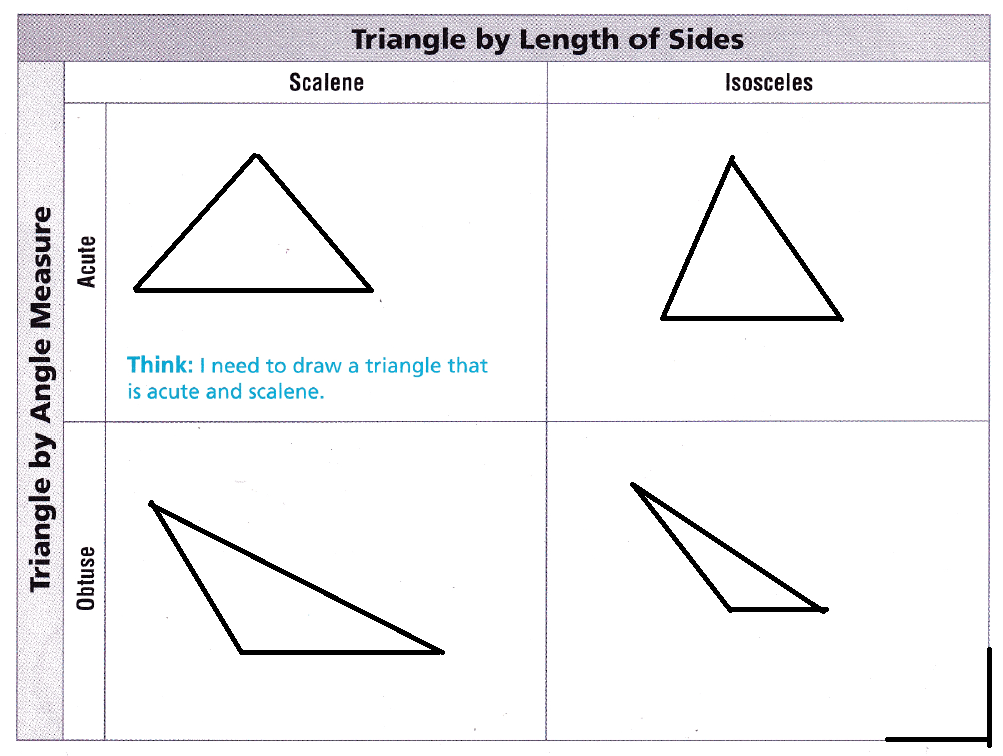Math Talk
Mathematical Processes
Can you draw a triangle that is right equilateral? Explain.
We already know that to be equilateral all the sides should be equal and all the corresponding angles also should be equal. So, it is not possible to draw a right-angled equilateral triangle

Share and Show

Classify each triangle. Write isosceles, scalene, or equilateral. Then write acute, obtuse, or right.

Question 1.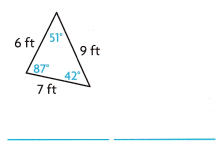The given triangle is: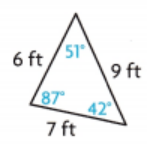Now,
From the given figure,
We can observe that
The side lengths are not equal
The angle measures are not equal
All the angle measures are less than 90°
Hence, from the above,
We can conclude that
The given triangle is a “Scalene Triangle” and an “Acute Triangle”

Question 2.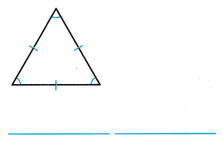The given triangle is: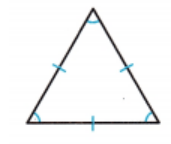Now,
From the given triangle,
We can observe that
All the side lengths are equal
All the angle measures are equal
All the angle measures are less than 90°
Hence, from the above,
We can conclude that
The given triangle is an “Equilateral Triangle” and an “Acute Triangle”

Question 3.The given triangle is: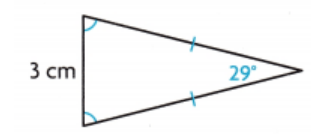Now,
From the given triangle,
We can observe that
The two side lengths are equal and one unequal side length
The two angle measures are equal
All the angle measures are less than 90°
Hence, from the above,
We can conclude that
The given triangle is an “Isosceles Triangle” and an “Acute Triangle”

Problem Solving

Question 4.
H.O.T. Can you tell that a triangle is obtuse, right, or acute without measuring the angles? Explain.
To determine whether the triangle is acute, right, or obtuse, add the squares of the two smaller sides and compare the sum to the square of the largest side. If this sum is greater, the triangle is acute, and if this sum is smaller, the triangle is obtuse

Question 5.
Multi-Step Draw 2 equilateral triangles that are congruent and share a side. What polygon is formed? Is it a regular polygon?
It is given that
Draw 2 equilateral triangles that are congruent and share a side
Now,
According to the given information,
The figure that can be drawn is:Now,
From the above polygon,
We can observe that
All the side lengths are equal
The angle measures are not the same
There are 4 sides
Hence, from the above,
We can conclude that
The polygon that is formed from the given information is: Quadrilateral
Since the angle measures are not the same, the polygon formed is not a “Regular Polygon”

Problem Solving

Question 6.
Analyze Shannon said that a triangle with exactly 2 congruent sides and an obtuse angle is an equilateral obtuse triangle. Describe her error.It is given that
Shannon said that a triangle with exactly 2 congruent sides and an obtuse angle is an equilateral obtuse triangle
Now,
We know that,
In an Equilateral triangle,
All three sides are equal and all the angle measures are also the same
In an Isosceles triangle,
Any two side lengths are the same and any two angle measures are the same
Hence, from the above,
We can conclude that
The error made by Shannon is:
The triangle is an “Obtuse Isosceles Triangle” but not the “equilateral Obtuse Triangle”

Classify the triangles in the structures below. Write isosceles, scalene, or equilateral. Then write acute, obtuse, or right.

Question 7.The given triangle is:Now,
From the given triangle,
The side lengths are different and the angle measures are acute
Hence, from the above,
We can conclude that
The given triangle is an “Acute Triangle” and a “Scalene triangle”

Question 8.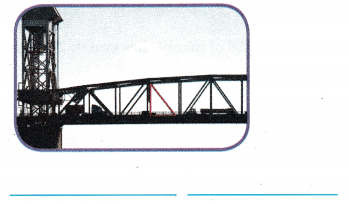The given triangle is:Now,
From the given triangle,
We can observe that
One of the angle measures is the “Right angle”
Hence, from the above,
We can conclude that
The given triangle is a “Right Triangle”

Question 9.
Ricky drew a triangle with angles measuring 90°, 30°, and 60°, and no congruent sides. Which of these describes the triangle?
(A) isosceles right
(B) equilateral obtuse
(C) scalene acute
(D) scalene right
It is given that
Ricky drew a triangle with angles measuring 90°, 30°, and 60°, and no congruent sides
Hence, from the above,
We can conclude thatQuestion 10.
Which of these triangles is isosceles and obtuse?We know that
An “Obtuse angle” is the measure that is greater than 90° and less than 180°
Hence, from the above,
We can conclude that
The triangle that is Isosceles and obtuse from the given triangles is: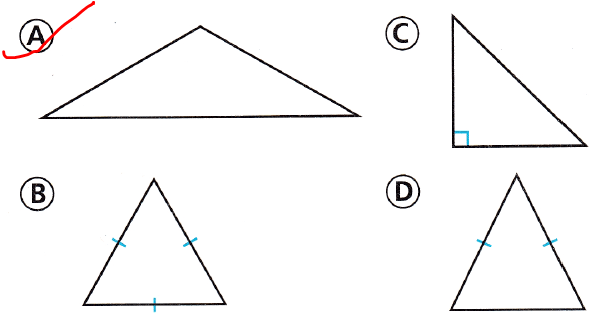Question 11.
Multi-Step Jenna drew this triangle and then drew the dotted line down the center. What type of triangle did she draw first? What triangles resulted from the dotted line?
(A) right; two scalene obtuse triangles
(B) equilateral; two right triangles
(C) scalene right; two right triangles
(D) isosceles acute; two equilateral trianglesIt is given that
Jenna drew this triangle and then drew the dotted line down the center
Now,
The given triangle is:Now,
From the given triangle,
We can observe that
The triangle is an “Equilateral triangle”
After the dotted line, there are 2 right triangles
Hence, from the above,
We can conclude that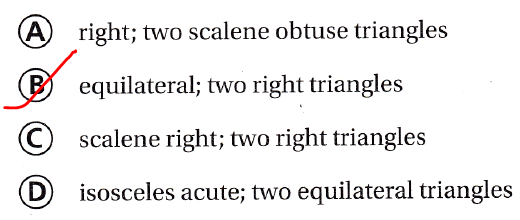Texas Test Prep

Question 12.
Which kind of triangle has exactly 2 congruent sides?
(A) isosceles
(B) equilateral
(C) scalene
(D) right
We know that,
The triangle that ahs exactly 2 congruent sides is: isosceles Triangle
Hence, from the above,
We can conclude that
The triangle that has exactly 2 congruent sides is:### Texas Go Math Grade 5 Lesson 11.2 Homework and Practice Answer Key

Classify each triangle. Write isosceles, scalene, or equilateral. Then write acute, obtuse, or right.

Question 1.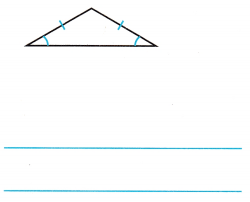The given triangle is: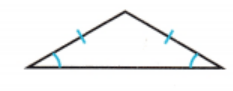Now,
From the given triangle,
We can observe that
There are 2 congruent sides and 2 congruent angles that are less than 90°
Hence, from the above,
We can conclude that
The given triangle is an ‘Isosceles triangle” and an “Acute Triangle”

Question 2.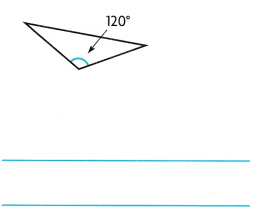The given triangle is: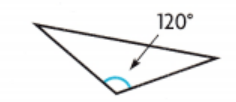Now,
From the given triangle,
We can observe that
One of the angles is an obtuse angle and all the side lengths are not equal
Hence, from the above,
We can conclude that
The given triangle is an “Obtuse Triangle” and a “Scalene Triangle”

Question 3.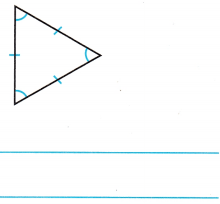The given triangle is: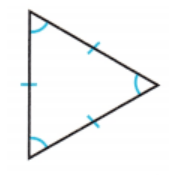Now,
From the given triangle,
We can observe that
All the side lengths are equal and all the angle measures are the same and less than 90°
Hence, from the above,
We can conclude that
The given triangle is an “Equilateral Triangle” and an “Acute Triangle”

Question 4.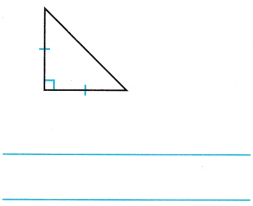The given triangle is: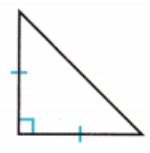Now,
From the given figure,
We can observe that
There are 2 congruent sides and 1 right angle
Hence, from the above,
We can conclude that
The given triangle is an “Isosceles triangle” and a “Right Triangle”

Question 5.The given triangle is: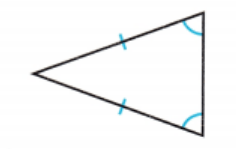From the given figure,
We can observe that
The two side lengths are congruent and the two angle measures are congruent
The angle measures are less than 90°
Hence, from the above,
We can conclude that
The given triangle is an “Isosceles triangle” and an “Acute Triangle”

Question 6.The given triangle is: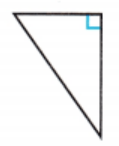Now,
From the given triangle,
We can observe that
One of the angles is  a right angle and all the side lengths are not the same
Hence, from the above,
We can conclude that
The given triangle is a “Scalene Triangle” and a “Right triangle”

Circle the figure that does not belong. Explain.

Question 7.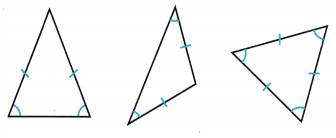The given figures are:Now,
From the given figures,
We can observe that
There are only 2 congruent sides in the first 2 figures and there are 3 congruent sides in the third figure
Hence, from the above,
We can conclude that
The figure that does not belong is: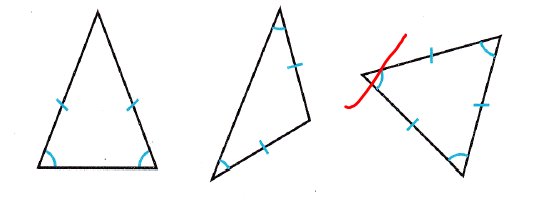Problem Solving

Question 8.
Parker drew a triangle with no congruent sides and a 95° angle. Classify the triangle by the lengths of its sides and measures of its angles. Explain.
It is given that
Parker drew a triangle with no congruent sides and a 95° angle
Now,
Since there are no congruent sides, the triangle is a “Scalene Triangle”
Now,
95° is an angle that is greater than 90° and less than 180° and this angle is called an “Obtuse angle”
Hence, from the above,
We can conclude that
The triangle is a “Scalene Triangle” since there are no congruent sides and is also called an “Obtuse Triangle” since one of the angles is 95°

Lesson Check

Question 9.
Lindsay draws a right triangle and adds the measures of the right angle and one acute angle. Which is a possible sum of the two angles?
(A) 180°
(B) 45°
(C) 90°
(D) 120°
It is given that
Lindsay draws a right triangle and adds the measures of the right angle and one acute angle
Now,
We know that,
The sum of the angles in a triangle is 180°
Now,
The sum of the angles is greater than 90° and less than 180°
Hence, from the above,
We can conclude that
The possible sum of the two angles is:Question 10.
Which of these triangles is isosceles and acute?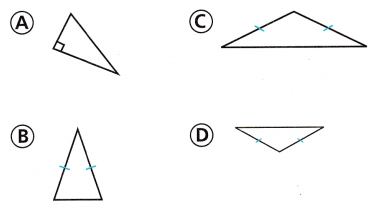We know that,
An “Isosceles triangle” is a triangle that has 2 congruent sides and 2 congruent angles and the angles less than 90°
Hence, from the above,
We can conclude that
The following triangles that are isosceles and acute are: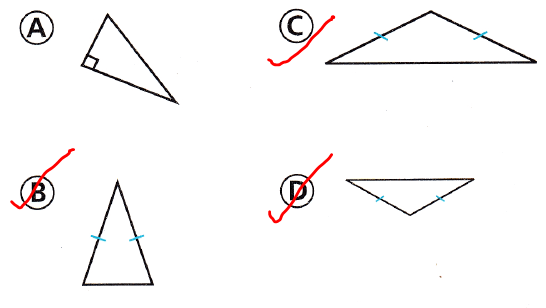Question 11.
Which kind of triangle has 3 congruent sides?
(A) right
(B) isosceles
(C) equilateral
(D) scalene
We know that,
The triangle that has 3 congruent sides is: Equilateral Triangle
Hence, from the above,
We can conclude that
The triangle that has 3 congruent sides is:Question 12.
Which of the following is another way to classify a scalene triangle with one angle greater than 90°?
(A) acute
(B) obtuse
(C) right
(D) equilateral
We know that,
The angle that is greater than 90°and less than 180° is an “Obtuse angle”
Hence, from the above,
We can conclude that
Another way to classify a scalene triangle with one angle greater than 90° is: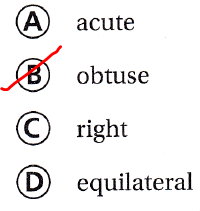Question 13.
Multi-Step Mrs. Bennett has a rug that is an equilateral triangle with a perimeter of 12 feet. Which could be the lengths of the sides of the rug?
(A) 4 ft, 4 ft, 4 ft
(B) 5 ft, 5 ft, 2 ft
(C) 3 ft, 3 ft, 3 ft
(D) 3 ft, 4 ft, 5 ft
It is given that
Mrs. Bennett has a rug that is an equilateral triangle with a perimeter of 12 feet
We know that,
The “Perimeter” is the sum of all the side lengths
In an equilateral triangle, all the side lengths are the same
So,
The perimeter of the equilateral triangle = 3 × Side length
3 × Side length = 12
Side length = $$\frac{12}{3}$$
Side length = 4 ft
Hence, from the above,
We can conclude that
The lengths of the sides of the rug are: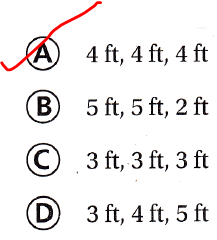Question 14.
Multi-Step Nathan cut a square tile in half for his kitchen floor design. He made one cut along a diagonal from one vertex to another vertex. Which triangles resulted from the cut?
(A) two scalene right triangles
(B) two isosceles obtuse triangles
(C) two isosceles right triangles
(D) two equilateral right triangles